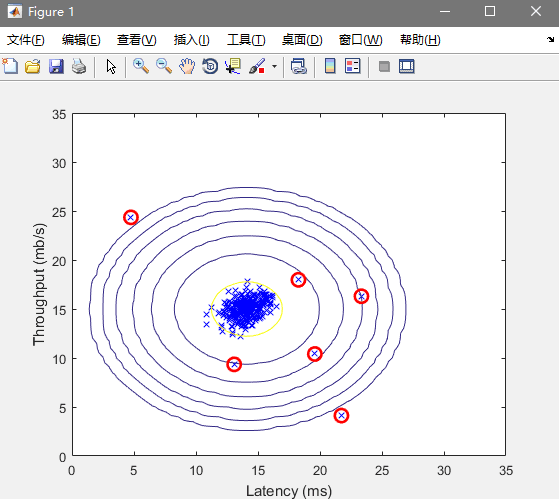吴恩达机器学习 - 异常检测

笔记：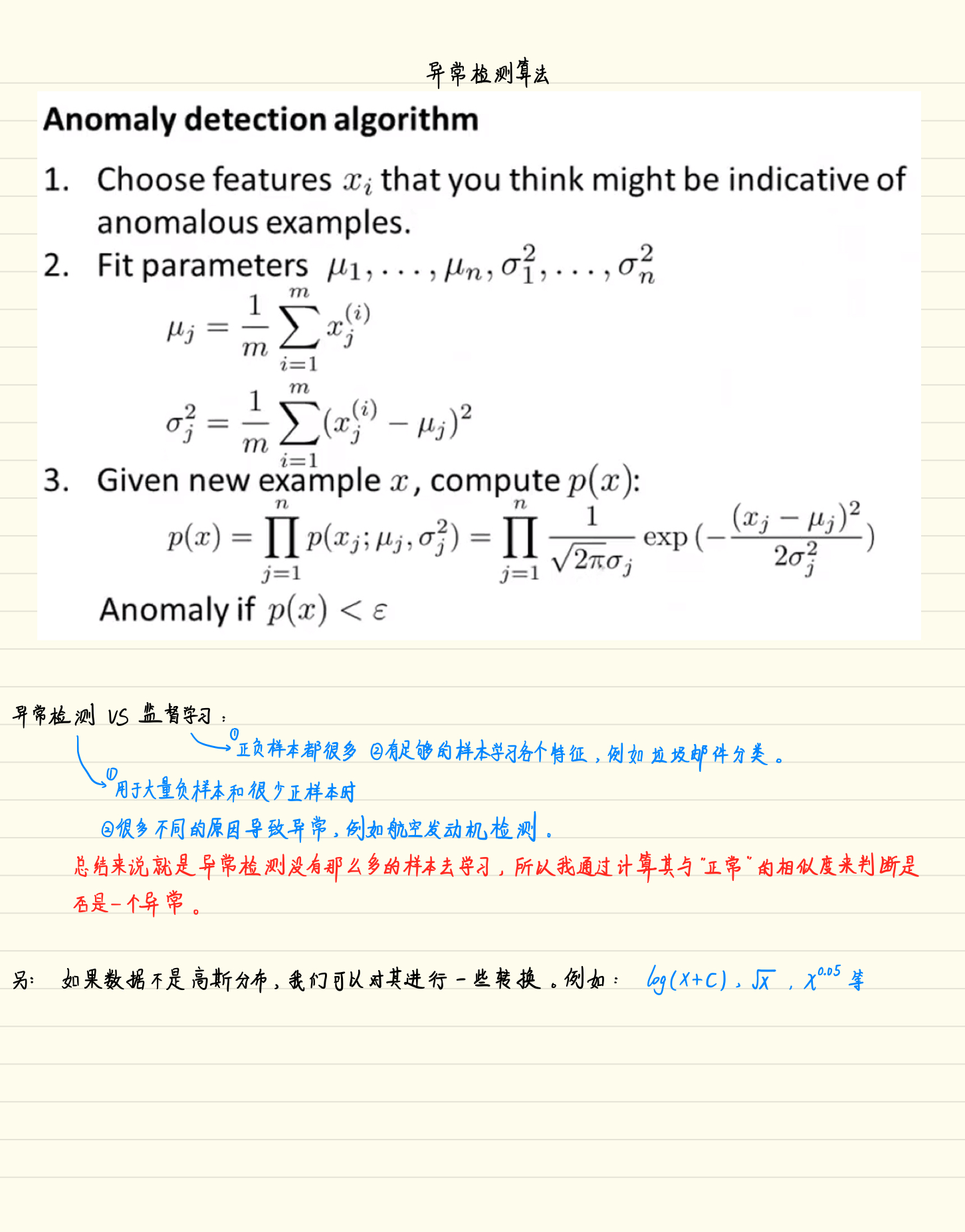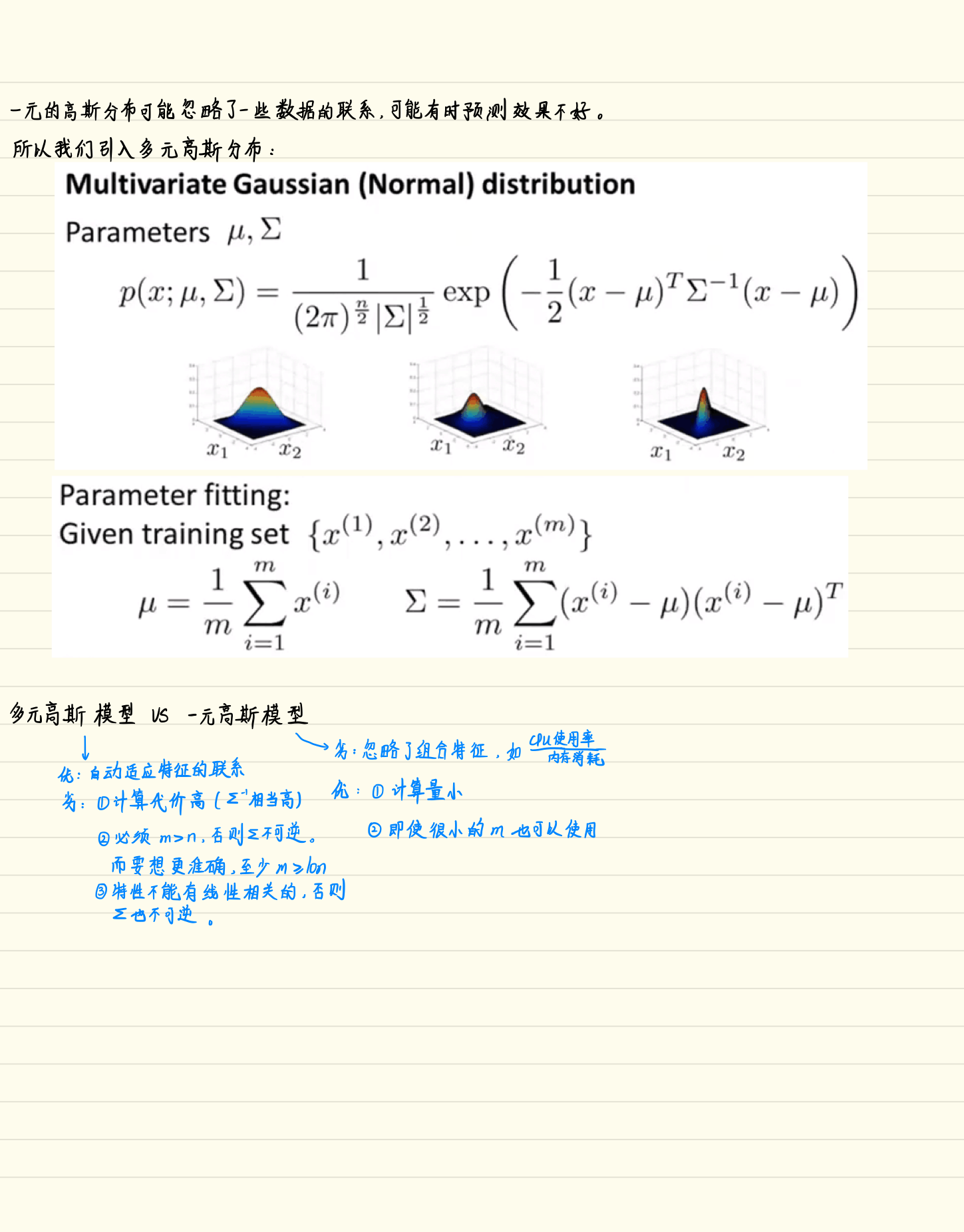数据可视化：

load('ex8data1.mat');

%  Visualize the example dataset
plot(X(:, 1), X(:, 2), 'bx');
axis([0 30 0 30]);
xlabel('Latency (ms)');
ylabel('Throughput (mb/s)');

效果图：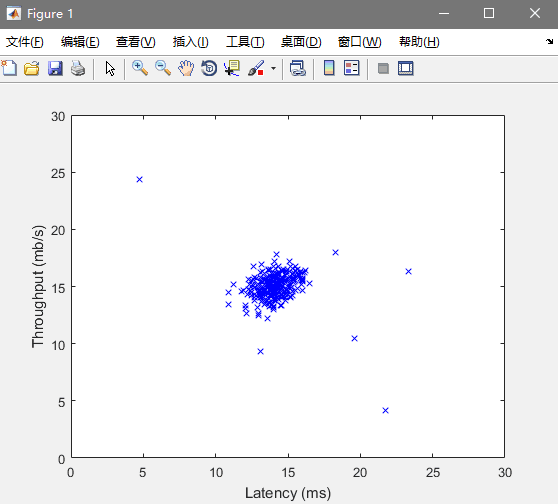求参数μ和σ2${\sigma }^{2}$$σ^{2}$：

公式：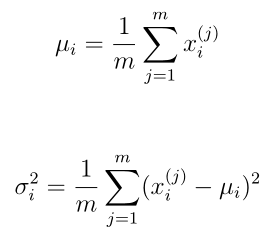Code（estimateGaussian.m）：

function [mu sigma2] = estimateGaussian(X)
%ESTIMATEGAUSSIAN This function estimates the parameters of a
%Gaussian distribution using the data in X
%   [mu sigma2] = estimateGaussian(X),
%   The input X is the dataset with each n-dimensional data point in one row
%   The output is an n-dimensional vector mu, the mean of the data set
%   and the variances sigma^2, an n x 1 vector
%

% Useful variables
[m, n] = size(X);

% You should return these values correctly
mu = zeros(n, 1);
sigma2 = zeros(n, 1);

% ====================== YOUR CODE HERE ======================
% Instructions: Compute the mean of the data and the variances
%               In particular, mu(i) should contain the mean of
%               the data for the i-th feature and sigma2(i)
%               should contain variance of the i-th feature.
%

mu = mean(X)';
sigma2 = (sum(bsxfun(@minus,X,mu').^2)./m)';

% =============================================================
end

选择最优的ε

这个用F1${F}_{1}$$F_{1}$来衡量：

公式：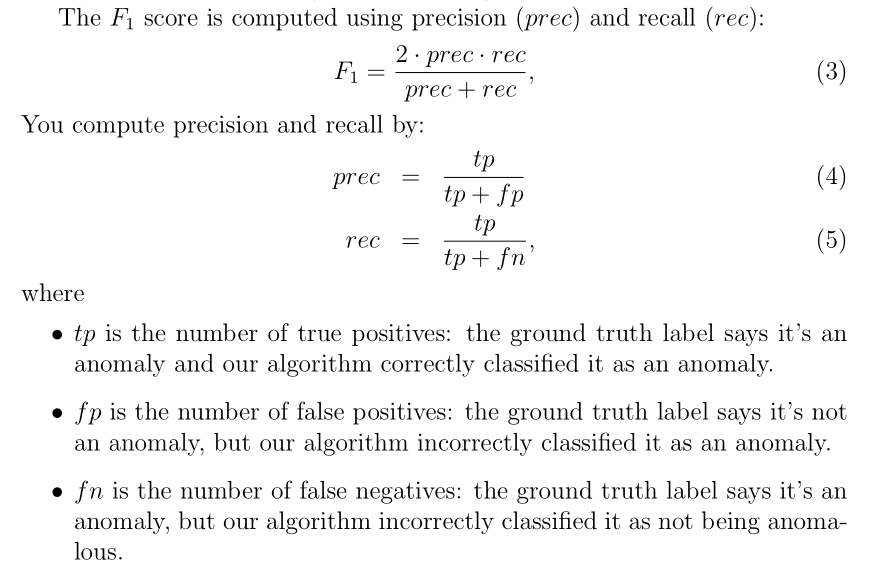Code（selectThreshold.m）（注意这里的true position,false position,false negative的求法）：
function [bestEpsilon bestF1] = selectThreshold(yval, pval)
%SELECTTHRESHOLD Find the best threshold (epsilon) to use for selecting
%outliers
%   [bestEpsilon bestF1] = SELECTTHRESHOLD(yval, pval) finds the best
%   threshold to use for selecting outliers based on the results from a
%   validation set (pval) and the ground truth (yval).
%

bestEpsilon = 0;
bestF1 = 0;
F1 = 0;

stepsize = (max(pval) - min(pval)) / 1000;
for epsilon = min(pval):stepsize:max(pval)

% ====================== YOUR CODE HERE ======================
% Instructions: Compute the F1 score of choosing epsilon as the
%               threshold and place the value in F1. The code at the
%               end of the loop will compare the F1 score for this
%               choice of epsilon and set it to be the best epsilon if
%               it is better than the current choice of epsilon.
%
% Note: You can use predictions = (pval < epsilon) to get a binary vector
%       of 0's and 1's of the outlier predictions

predictions = pval < epsilon;
tp = sum((predictions == 1) & (yval == 1));     %true positive
fp = sum((predictions == 1) & (yval == 0));     %false positive
fn = sum((predictions == 0) & (yval == 1));     %false negative
prec = tp/(tp+fp);      %precision
rec = tp/(tp+fn);       %recall
F1 = 2*prec*rec/(prec+rec);

% =============================================================

if F1 > bestF1
bestF1 = F1;
bestEpsilon = epsilon;
end
end

end

效果图（求出ε后，继续运行ex8.m画出的图）：